# Hot & Cold Therapy

Grid List
\$21.10
Increase value Decrease value
\$100.87
Increase value Decrease value
\$100.87
Increase value Decrease value
\$100.87
Increase value Decrease value
\$230.00
Increase value Decrease value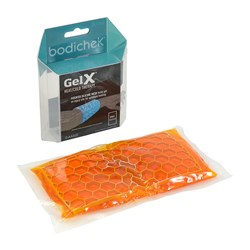\$12.99
Increase value Decrease value
\$713.90
Increase value Decrease value
P.O.A.
\$1452.00
Increase value Decrease value
\$7.22
Increase value Decrease value
\$6.49
Increase value Decrease value
\$27.94
Increase value Decrease value
\$19.57
Increase value Decrease value
\$7.33
Increase value Decrease value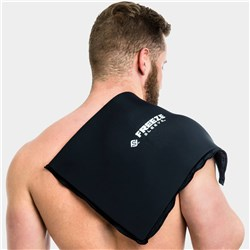\$99.95
Increase value Decrease value
\$406.85
Increase value Decrease value
\$178.00
Increase value Decrease value
\$180.00
Increase value Decrease value
\$96.00
Increase value Decrease value
Aircast Cryo Cuff - Elbow Cuff
\$178.00
Increase value Decrease value
\$28.35
Increase value Decrease value
\$24.05
Increase value Decrease value
\$24.30
Increase value Decrease value
\$6.53
Increase value Decrease value
\$63.90
Increase value Decrease value
\$1.99
Increase value Decrease value
\$1.99
Increase value Decrease value
\$32.00
Increase value Decrease value
\$66.00
Increase value Decrease value
Hydrocollator Hotpac Standard
\$28.80
Increase value Decrease value
\$48.00
Increase value Decrease value
\$49.60
Increase value Decrease value
\$7.70
Increase value Decrease value
\$21.70
Increase value Decrease value
\$85.47
Increase value Decrease value
Lumark Shoulder Cold Compression
\$109.00
Increase value Decrease value
\$58.99
Increase value Decrease value
\$50.90
Increase value Decrease value
\$39.95
Increase value Decrease value
\$199.00
Increase value Decrease value
From
Increase value Decrease value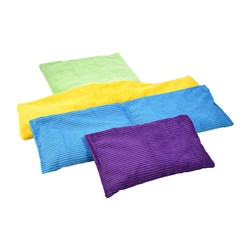From
Increase value Decrease value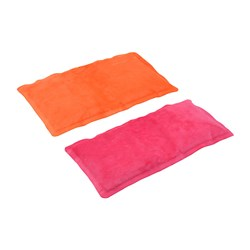From
Increase value Decrease value
From
Increase value Decrease value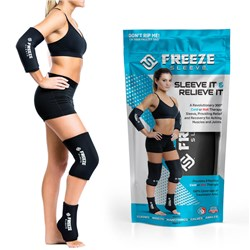From
Increase value Decrease value
From
Increase value Decrease value
From
Increase value Decrease value
From
Increase value Decrease value
Grid List
##### Products to compare:
Comparing Products